# Structural modelIntroduction >
The structural frame
The construction
The reinforcement I
The reinforcement II
Quantity/Cost estimation
Detailing drawingsIntroduction >
Wind and Seismic Forces >
Structural model and Analysis
Modelling slabs
Slabs
Seismic behavour of frames
Appendix A
Appendix B
Appendix C
Appendix DIntroduction >
Materials
To be continued >

## Effective span of beams and slabs [EC2 §5.3.2.2]leff=lnleftright  where α=min(t/2,h/2)

·         At the end support of the first slab, the width is considered to be a=t1/2=125 mm which is acceptable as in favor of the safety.

·         The width of beam-column supports, is taken into account more efficiently, when the analysis uses rigid bodies (see next paragraph).

## Effective flange width of continuous beam [EC2 §5.3.2.1]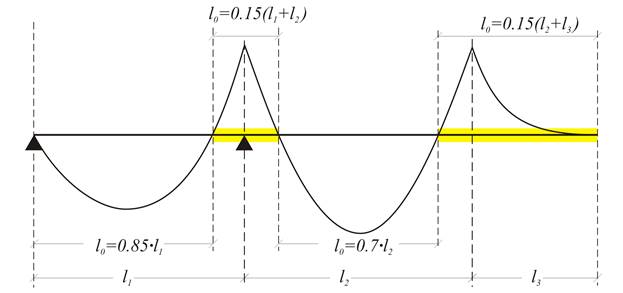Continuous beam: lo distance between consecutive points of zero moments

## Effective flange width of continuous frame beamContinuous frame beam: lo distance between consecutive points of zero moments

Earthquake resistant structures require strong columns and fixed column-beam connections. This requirement demands the creation of a frame set of beams, forming a continuous structure with respect to geometry, but autonomous with respect to the adjacent beams. This fact leads to the conclusion that, in general, the supports of a beam are rarely pinned. Therefore, lo=0.70×l can be chosen for all the earthquake resistant beams.

## Effective flange width parameters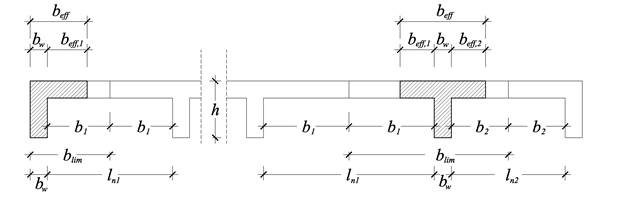beff=bw+beff,1+beff,2≤blim where beff,1=0.20×b1+0.10×lo≤0.20×lo and beff,2=0.20×b2+0.10×lo≤0.20×lo.

·         Τhe effective widths at supports have practical meaning mainly for the dimensioning of  inverted concrete beams under bending.

·         When an adjacent slab is cantilever, of a span ln, the corresponding b1 or b2 is equal to ln.

## Rigid bodies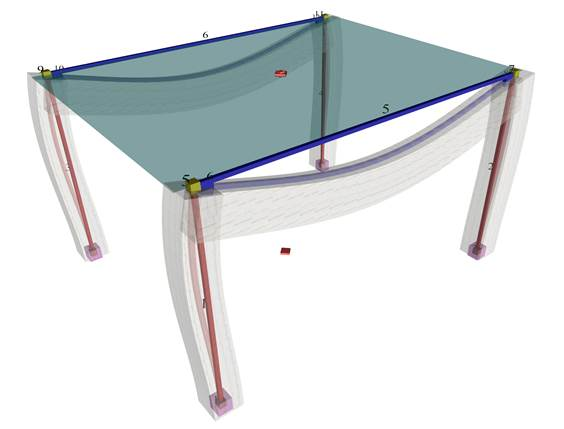The actual structure and the space model (screen shot captured by HoloBIM)

The space frame model comprises nodes (e.g. node 1, 5, 9 etc.) and members (e.g. member 1, 2, 5 etc.). Nodes are symbolic geometric points where members end.

In general, each node has 6 displacements (6 degrees of freedom), three translations δx, δy, δz and three rotations φx, φy, φz. The objective of the analysis is the calculation of the 6 displacements for all nodes.

## Detail of rigid body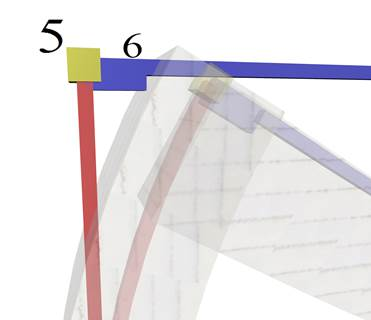Plan and 3D of column-beam nodes: Master node (5) and slave node (6)

Provided the 6 displacements of the master node are δxδyδzφxφyφz, the corresponding δx,sδy,sδz,sφx,sφy,sφz,s of the slave node are:

φ x,s = φ x , φ y,s = φ y , φ z,s = φ z , δ x,s = δ x -sy × φ (given sz=0), δ y,s = δ y +sx × φ (given sz=0),

δ z,s = δ z -sx × φ y +sy × φ x

## Diaphragmatic behaviour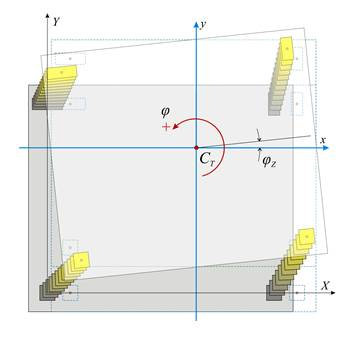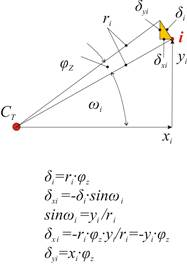Diaphragmatic behaviour of floor and the displacements of a random point i of the diaphragm due to φz

The 3 displacements δzφxφy of each node belonging to the diaphragm are independent of each other, while the rest δxδyφz are depended on the 3 displacements of point CT called Center of Elastic Torsion of the diaphragm. Displacements δxiδyiφzi at the point i of the horizontal diaphragm are expressed as:

φzi=φz, δxi=δxCT-yi×φz, δyi=δyCT+xi×φz